Next: Example 2 Up: Numerical examples Previous: Numerical examples

## Example 1

In this example we choose a nonlinear partial differential equation and homogeneous Dirichlet boundary conditions: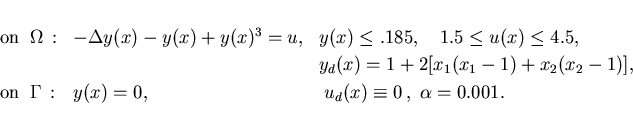These equations are related to a simplified Ginzburg-Landau model for super-con- ductivity in the absence of internal magnetic fields with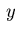the wave function; cf. Ito, Kunisch  and Kunisch, Volkwein .

The adjoint equations (2.10), (2.12) apply: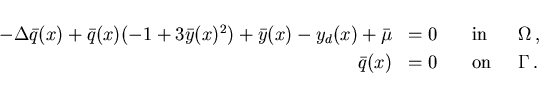(4.1)

Since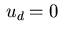, the minimum condition (2.21) yields the control law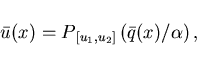(4.2)

where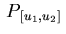denotes the projection operator onto the interval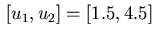. The optimal control is shown in Figure 1, while Figure 2 depicts the optimal state and associated adjoint variable. The adjoint variable permits the verification of the control law (4.2). The state is active in the center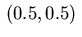. Figure 2 indicates that condition (2.7) is not satisfied since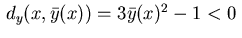holds for all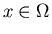. However, well-posedness of the Ginzburg-Landau model follows from results in Gunzburger et al. .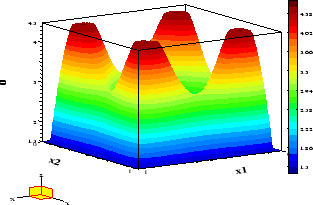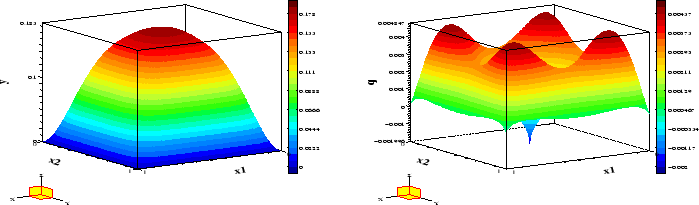Table 1: Information on solution of Example 1
 N+1 it CPU Acc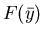50 26 104 8 .0577903 100 30 1897 8 .0621615 200 35 54831 8 .0644259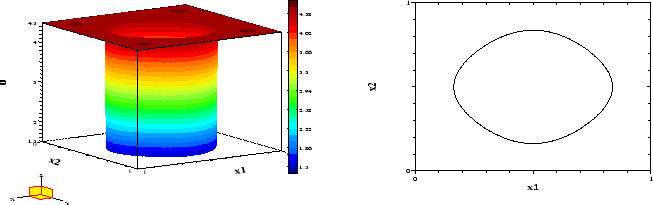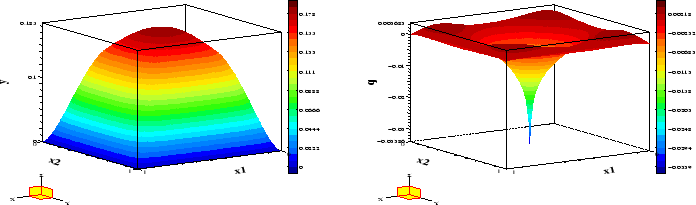Next: Example 2 Up: Numerical examples Previous: Numerical examples
Hans D. Mittelmann
2000-10-06